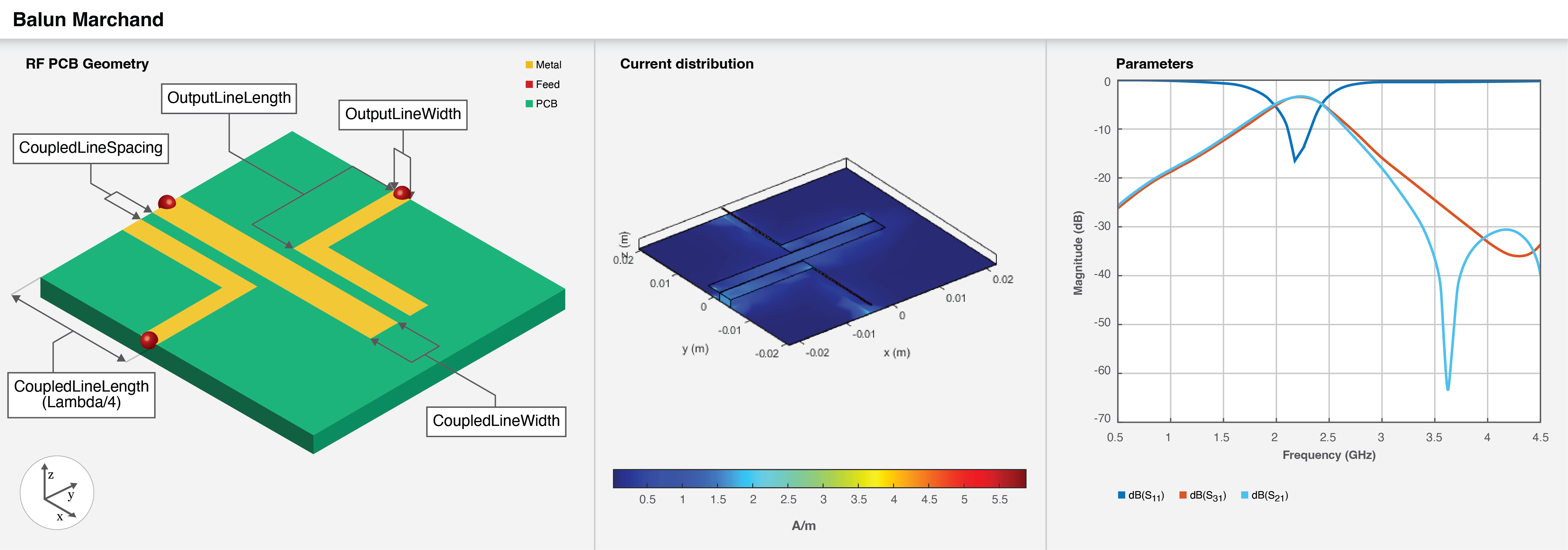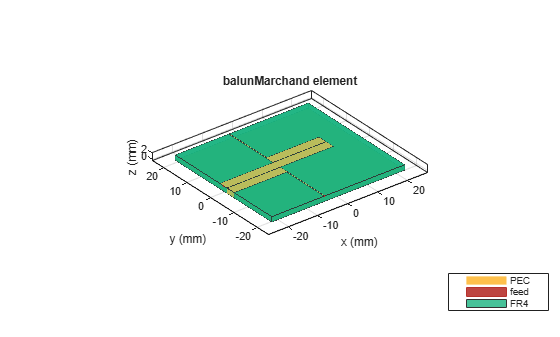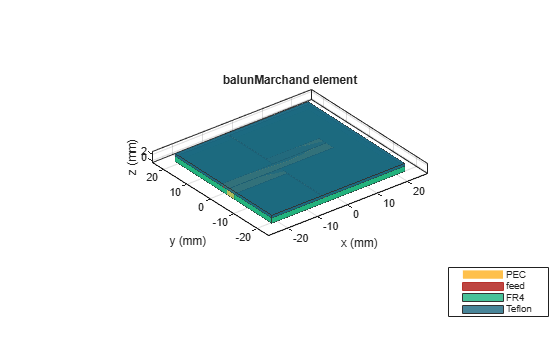# balunMarchand

Create Marchand balun in microstrip form

Since R2022b

## Description

Use the `balunMarchund` object to create a Marchand balun in the microstrip form with an unbalanced and balanced output. There is a 180 degrees phase difference between the input and the output ports.Baluns are used to connect an unbalanced source line to a balanced load or vice-versa enabling you to create a matching transition between balanced and unbalanced transmission lines. A Marchand balun has a pair of quarter-wavelength coupled lines of quarter wavelength for dividing and combining signals with the same amplitude and opposite phase.

## Creation

### Syntax

``balun = balunMarchand``
``balun = balunMarchand(Name=Value)``

### Description

example

````balun = balunMarchand` creates a Marchand balun in the microstrip form with default properties for a resonant frequency of 2.2 GHz.```

example

````balun = balunMarchand(Name=Value)` sets Properties using one or more name-value arguments. For example, `balunMarchand(OutputLineLength=0.016)` creates a Marchand balun with an output line length of `0.016` meters. Properties not specified retain their default values.```

## Properties

expand all

Length of the coupled line in meters, specified as a positive scalar.

Example: `balun = balunMarchand(CoupledLineLength=0.0254)`

Data Types: `double`

Width of the coupled line in meters, specified as a positive scalar.

Example: `balun = balunMarchand(CoupledLineWidth=0.0005)`

Data Types: `double`

Spacing between the coupled lines in meters, specified as a positive scalar.

Example: `balun = balunMarchand(CoupledLineSpacing=0.00014)`

Data Types: `double`

Length of the output line in meters, specified as a positive scalar.

Example: `balun = balunMarchand(OutputLineLength=0.0224)`

Data Types: `double`

Width of the output line in meters, specified as a positive scalar.

Example: `balun = balunMarchand(OutputLineWidth=0.000253)`

Data Types: `double`

Height of the Marchand balun from the ground plane in meters, specified as a positive scalar.

In the case of a multilayer substrate, you can use the `Height` property to create a Marchand balun where the two dielectrics interface.

Example: `balun = balunMarchand(Height=0.022)`

Data Types: `double`

Type of dielectric material used as a substrate, specified as a `dielectric` object. The default height of the substrate is `0.0013` meters. The dielectric material in a `balunMarchand` object with default properties is FR4.

Example: `d = dielectric("RTDuriod");` `balun =balunMarchand(Substrate=d)`

Type of metal used in the conducting layers, specified as a `metal` object. The type of metal in a `balunMarchand` object with default properties is PEC.

Example: `m = metal("Copper");` `balun =balunMarchand(Conductor=m)`

Data Types: `string` | `char`

## Object Functions

 `charge` Calculate and plot charge distribution `current` Calculate and plot current distribution `designCoupledLine` Calculate dimensions of coupled-line section for specified frequency `designUncoupledLine` Calculate dimensions of uncoupled-line section for specified frequency `designOutputLine` Calculate dimensions of output line section for specified frequency `feedCurrent` Calculate current at feed port `layout` Plot all metal layers and board shape `mesh` Change and view mesh properties of metal or dielectric in PCB component `shapes` Extract all metal layer shapes of PCB component `show` Display PCB component structure or PCB shape `sparameters` Calculate S-parameters for RF PCB objects

## Examples

collapse all

Create a default Marchand balun.

`balun = balunMarchand`
```balun = balunMarchand with properties: CoupledLineLength: 0.0178 CoupledLineWidth: 0.0030 CoupledLineSpacing: 1.5000e-04 OutputLineLength: 0.0160 OutputLineWidth: 2.9000e-04 Height: 0.0016 Substrate: [1x1 dielectric] Conductor: [1x1 metal] ```

View the balun.

`show(balun)`Create a Marchand balun.

```balun = balunMarchand; balun.Height = 0.0016;```

Create a multilayer dielectric of FR4 and Teflon.

```sub = dielectric('FR4','Teflon'); sub.Thickness =[0.0016 0.0008]; balun.Substrate = sub; figure;```

View the balun.

`show(balun);`Pozar, David M. Microwave Engineering. 4th ed. Hoboken, NJ: Wiley, 2012.